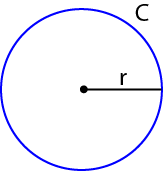## Ultimate Circle area circumference radius calculator

Input >>> Is This Number

Then a Circle has:

Significant Figures >>>

The circle calculator could help you find the area, circumference or radius of a circle. If you know one of the variable of a circle you can calculate the other 3 unknowns.

Area of a circle: A = πr2 = πd2/4

Circumference of a circle: C = 2πr = πd

Where, r = radius, C = circumference, A = area, π = pi = 3.14159Chord, secant, tangent, radius, and diameter

Thinkcalculator.com provides you helpful and handy calculator resources.